# Grouped Aggregate Pushdown

The SQLPerformance.com bi-weekly newsletter keeps you up to speed on the most recent blog posts and forum discussions in the SQL Server community.

eNews is a bi-monthly newsletter with fun information about SentryOne, tips to help improve your productivity, and much more.

Subscribe

Featured AuthorErin Stellato is a Principal Consultant with SQLskills and a Microsoft Data Platform MVP.

Erin’s Posts

## Introduction

In SQL Server 2012, grouped (vector) aggregation was able to use parallel batch-mode execution, but only for the partial (per-thread) aggregate. The associated global aggregate always ran in row mode, after a Repartition Streams exchange.

SQL Server 2014 added the ability to perform parallel batch-mode grouped aggregation within a single Hash Match Aggregate operator. This eliminated unnecessary row-mode processing, and removed the need for an exchange.

SQL Server 2016 introduced serial batch mode processing and aggregate pushdown. When pushdown is successful, aggregation is performed within the Columnstore Scan operator itself, possibly operating directly on compressed data, and taking advantage of SIMD CPU instructions.

The performance improvements possible with aggregate pushdown can be very substantial. The documentation lists some of the conditions required to achieve pushdown, but there are cases where the lack of ‘locally aggregated rows’ cannot be fully explained from those details alone.

This article covers additional factors that affect aggregate pushdown for `GROUP BY` queries only. Scalar aggregate pushdown (aggregation without a `GROUP BY` clause), filter pushdown, and expression pushdown may be covered in a future post.

## Columnstore storage

The first thing to say is that aggregate pushdown only applies to compressed data, so rows in a delta store are not eligible. Beyond that, pushdown can depend on the type of compression used. To understand this, it is necessary to first review how columnstore storage works at a high level:

A compressed row group contains a column segment for each column. The raw column values are encoded in a 4-byte or 8-byte integer using value or dictionary encoding.

Value encoding can reduce the number of bits required for storage by translating raw values using a base offset and magnitude modifier. For example, the values {1100, 1200, 1300} can be stored as (0, 1, 2) by first scaling by a factor of 0.01 to give {11, 12, 13}, then rebasing at 11 to give {0, 1, 2}.

Dictionary encoding is used when there are duplicate values. It can be used with non-numeric data. Each unique value is stored in a dictionary and assigned an integer id. The segment data then references id numbers in the dictionary instead of the original values.

After encoding, segment data may be further compressed using run-length encoding (RLE) and bit-packing:

RLE replaces repeating elements with the data and the number of repeats, for example {1, 1, 1, 1, 1, 2, 2, 2} could be replaced with {5×1, 3×2}. RLE space savings increase with the length of the repeating runs. Short runs can be counterproductive.

Bit-packing stores the binary form of the data in as narrow a common window as possible. For example, the numbers {7, 9, 15} are stored in binary (single-byte for space) integers as {00000111, 00001001, 00001111}. Packing these bits into a fixed four-bit window gives the stream {011110011111}. Knowing there is a fixed window size means there is no need for a delimiter.

Encoding and compression are separate steps, so RLE and bit-packing are applied to the result of value-encoding or dictionary-encoding the raw data. Further, data within the same column segment can have a mixture of RLE and bit-packing compression. RLE-compressed data is called pure, and bit-packed compressed data is called impure. A column segment may contain both pure and impure data.

The space savings that can be achieved through encoding and compression may depend on ordering. All column segments within a row group must be implicitly sorted the same way so SQL Server can efficiently reconstruct complete rows from the column segments. Knowing that row 123 is stored at the same position (123) in each column segment means the row number does not have to be stored.

One downside to this arrangement is that a common sort order has to be chosen for all column segments in a row group. A particular ordering might suit one column very well, but miss significant opportunities in other columns. This is most clearly the case with RLE compression. SQL Server uses Vertipaq technology to determine a good way of sorting columns in each row group to give a good overall compression result.

SQL Server currently only uses RLE within a column segment when there is a minimum of 64 contiguous repeating values. The remaining values in the segment are bit-packed. As noted, whether repeating values appear as contiguous in a column segment depends on the ordering chosen for the row group.

SQL Server supports specialized SIMD bit unpacking for bit widths from 1 to 10 inclusive, 12, and 21 bits. SQL Server can also use standard integer sizes e.g. 16, 32, and 64 bits with bit-packing. These numbers are chosen because they fit nicely in a 64-bit unit. For example, one unit can hold three 21-bit subunits, or 5 12-bit subunits. SQL Server does not cross a 64-bit boundary when packing bits.

SIMD uses 256-bit registers when the processor supports AVX2 instructions, and 128-bit registers when SSE4.2 instructions are available. Otherwise, non-SIMD unpacking can be used.

## Grouped aggregate pushdown conditions

Most plans with a Hash Match Aggregate operator directly above a Columnstore Scan operator will potentially qualify for grouped aggregate pushdown, subject to the general conditions noted in the documentation.

Extra filters and expressions can also sometimes be added without preventing grouped aggregate pushdown. The general rule is that the filter or expression must also be capable of pushdown (though compatible expressions may still appear in a separate Compute Scalar). As noted in the introduction, these aspects may be covered in detail in separate articles.

There is currently nothing in execution plans to indicate whether a particular aggregate was considered generally compatible with grouped aggregate pushdown or not. Still, when the plan generally qualifies for grouped aggregate pushdown, both pushdown (fast) and non-pushdown (slow) code paths are made available.

Each scan output batch (of up to 900 rows) makes a runtime decision between the fast and slow code paths. This flexibility allows as many batches as possible to benefit from pushdown. In the worst case, no batches will use the fast path at runtime, despite a ‘generally compatible’ plan.

The execution plan shows the result of fast-path pushdown processing as ‘locally aggregated rows’ with no corresponding row output from the scan. Slow-path batches appear as output rows from the columnstore scan as usual, with the aggregation performed by a separate operator instead of at the scan.

A single grouped aggregate and scan combination can send some batches down the fast path and some down the slow path, so it is perfectly possible to see some, but not all, rows locally aggregated. When grouped aggregate pushdown is successful, each output batch from the scan contains grouping keys and a partial aggregate representing the rows that contributes.

### Detailed checks

There are a number of runtime checks to determine if pushdown processing can be used. Among the lightly documented checks are:

• There must be no possibility of aggregate overflow.
• Any impure (bit-packed) grouping keys must be no wider than 10 bits. Pure (RLE encoded) grouping keys are treated as having an impure width of zero, so these usually present few obstacles.
• Pushdown processing must continue to be considered worthwhile, using a ‘benefit measure’ updated at the end of each output batch.

The possibility of aggregate overflow is conservatively assessed for each batch based on the type of aggregate, result data type, current partial aggregation values, and information about the input data. For example, SQL Server knows minimum and maximum values from segment metadata as exposed in the DMV `sys.column_store_segments`. Where there is a risk of overflow, the batch will use slow path processing. This is mostly a risk for the `SUM` aggregate.

The restriction on impure grouping key width is worth emphasising. It only applies to columns in the `GROUP BY` clause that are actually used in the execution plan as a basis for grouping. These sets are not always exactly the same because the optimizer has freedom to remove redundant grouping columns, or to otherwise rewrite aggregates, so long as the final query results are guaranteed to match the original query specification. Where there is a disparity, it is the grouping columns shown in the execution plan that matter.

The bigger difficulty is knowing whether any of the grouping columns is stored using bit-packing, and if so, what width was used. It would also be useful to know how many values were encoded using RLE. This information could be in the `column_store_segments` DMV, but that is not the case today. As far as I know, there is no documented way right now to get bit-packing and RLE information from metadata. That leaves us with looking for undocumented alternatives.

### Finding RLE and bit-packing information

The undocumented `DBCC CSINDEX` can give us the information we need. Trace flag 3604 needs to be on for this command to produce output in the SSMS messages tab. Given information about the column segment we are interested in, this command returns:

• Segment attributes (similar to `column_store_segments`)
• RLE information
• Bookmarks into RLE data
• Bitpack information

Being undocumented, there are a few quirks (such as having to add one to column ids for clustered columnstore, but not nonclustered columnstore), and even a couple of minor errors. You should not use it on anything except a personal test system. Hopefully, one day a supported method to access this data will be provided instead.

## Examples

The best way to show `DBCC CSINDEX` and demonstrate the points made so far in this text is to work through some examples. The scripts that follow assume there is a table called `dbo.Numbers` in the current database that contains integers from 1 to at least 16,384. Here is a script to create my standard version of this table with ten million integers:

```IF OBJECT_ID(N'dbo.Numbers', N'U') IS NOT NULL
BEGIN
DROP TABLE dbo.Numbers;
END;
GO
WITH Ten(N) AS
(
SELECT 1 UNION ALL SELECT 1 UNION ALL
SELECT 1 UNION ALL SELECT 1 UNION ALL
SELECT 1 UNION ALL SELECT 1 UNION ALL
SELECT 1 UNION ALL SELECT 1 UNION ALL
SELECT 1 UNION ALL SELECT 1
)
SELECT
n = IDENTITY(int, 1, 1)
INTO dbo.Numbers
FROM Ten AS T10
CROSS JOIN Ten AS T100
CROSS JOIN Ten AS T1000
CROSS JOIN Ten AS T10000
CROSS JOIN Ten AS T100000
CROSS JOIN Ten AS T1000000
CROSS JOIN Ten AS T10000000
ORDER BY n
OFFSET 0 ROWS
FETCH FIRST 10 * 1000 * 1000 ROWS ONLY
OPTION
(MAXDOP 1);
GO
ALTER TABLE dbo.Numbers
PRIMARY KEY CLUSTERED (n)
WITH
(
SORT_IN_TEMPDB = ON,
MAXDOP = 1,
FILLFACTOR = 100
);```

The examples all use the same basic test table: The first column `c1` contains a unique number for each row. The second column `c2` is populated with a number of duplicates for each of a small number of distinct values.

A clustered columnstore index is created after data population so that all of the test data ends up in a single compressed row group (no delta store). It is built replacing a b-tree clustered index on column `c2` to encourage the VertiPaq algorithm to consider the usefulness of sorting on that column early on. This is the basic test setup:

```USE Sandpit;
GO
DROP TABLE IF EXISTS dbo.Test;
GO
CREATE TABLE dbo.Test
(
c1 integer NOT NULL,
c2 integer NOT NULL
);
GO
DECLARE
@values integer = 512,
@dupes integer = 63;

INSERT dbo.Test
(c1, c2)
SELECT
N.n,
N.n % @values
FROM dbo.Numbers AS N
WHERE
N.n BETWEEN 1 AND @values * @dupes;
GO
-- Encourage VertiPaq
CREATE CLUSTERED INDEX CCSI ON dbo.Test (c2);
GO
CREATE CLUSTERED COLUMNSTORE INDEX CCSI
ON dbo.Test
WITH (MAXDOP = 1, DROP_EXISTING = ON);```

The two variables are for the number of distinct values to insert in column `c2`, and the number of duplicates for each of those values.

The test query is a very simple grouped `COUNT_BIG` aggregation using column `c2` as the key:

```-- The test query
SELECT
T.c2,
numrows = COUNT_BIG(*)
FROM dbo.Test AS T
GROUP BY
T.c2;```

Columnstore index information will be displayed using `DBCC CSINDEX` after each test query execution:

```DECLARE
@dbname sysname = DB_NAME(),
@objectid integer = OBJECT_ID(N'dbo.Test', N'U');

DECLARE
@rowsetid bigint =
(
SELECT
P.hobt_id
FROM sys.partitions AS P
WHERE
P.[object_id] = @objectid
AND P.index_id = 1
AND P.partition_number = 1
),
@rowgroupid integer = 0,
@columnid integer =
COLUMNPROPERTY(@objectid, N'c2', 'ColumnId') + 1;

DBCC CSINDEX
(
@dbname,
@rowsetid,
@columnid,
@rowgroupid,
1, -- show segment data
2, -- print option
0, -- start bitpack unit (inclusive)
2 -- end bitpack unit (exclusive)
);```

Tests were run on the latest released version of SQL Server available at the time of writing: Microsoft SQL Server 2017 RTM-CU13-OD build 14.0.3049 Developer Edition (64-bit) on Windows 10 Pro. Things should work just fine on the latest build of SQL Server 2016 as well.

## Test 1: Pushdown, 9-bit Impure Keys

This test uses the test data population script exactly as written just above, producing a table with 32,256 rows. Column `c1` contains numbers from 1 to 32,256.

Column `c2` contains 512 distinct values from 0 to 511 inclusive. Each value in `c2` is duplicated 63 times, but they do not appear as contiguous blocks when viewed in `c1` order; they cycle 63 times through values 0 to 511.

Given the foregoing discussion, we expect SQL Server to store the `c2` column data using:

• Dictionary encoding since there are a significant number of duplicated values.
• No RLE. The number of duplicates (63) per value does not reach the threshold of 64 required for RLE.
• Bit-packing size 9. The 512 distinct dictionary entries will exactly fit in 9 bits (2^9 = 512). Each 64-bit unit will contain up to seven 9-bit subunits.

This is all confirmed as correct using the `DBCC CSINDEX` query:

The Segment Attributes section of the output shows dictionary encoding (type 2; the values for `encodingType` are as documented at `sys.column_store_segments`).

Segment Attributes:

Version = 1 encodingType = 2 hasNulls = 0
BaseId = -1 Magnitude = -1.000000e+000 PrimaryDictId = 0
SecondaryDictId = -1 MinDataId = 0 MaxDataId = 511
NullValue = -1 OnDiskSize = 37944 RowCount = 32256

The RLE section shows no RLE data, only a pointer to the bit-packed region, and an empty entry for value zero:

Lob type = 3 RLE Array Count (In terms of Native Units) = 2
RLE Array Entry Size = 8
RLE Data:

Index = 0 Bitpack Array Index = 0 Count = 32256
Index = 1 Value = 0 Count = 0

The Bitpack Data Header section shows bitpack size 9 and 4,608 bitpack units used:

Bitpack Entry Size = 9 Bitpack Unit Count = 4608 Bitpack MinId = 3
Bitpack DataSize = 36864

The Bitpack Data section shows the values stored in the first two bitpack units as requested by the last two parameters to the `DBCC CSINDEX` command. Recall that each 64-bit unit can hold 7 subunits (numbered 0 to 6) of 9 bits each (7 x 9 = 63 bits). The 4,608 units overall hold 4,608 * 7 = 32,256 rows:

Bitpack Data:

Unit 0 SubUnit 0 = 383
Unit 0 SubUnit 1 = 255
Unit 0 SubUnit 2 = 127
Unit 0 SubUnit 3 = 510
Unit 0 SubUnit 4 = 381
Unit 0 SubUnit 5 = 253
Unit 0 SubUnit 6 = 125

Unit 1 SubUnit 0 = 508
Unit 1 SubUnit 1 = 379
Unit 1 SubUnit 2 = 251
Unit 1 SubUnit 3 = 123
Unit 1 SubUnit 4 = 506
Unit 1 SubUnit 5 = 377
Unit 1 SubUnit 6 = 249

Since the grouping keys use bit-packing with a size less than or equal to 10, we expect grouped aggregate pushdown to work here. Indeed, the execution plan shows all rows were locally aggregated at the Columnstore Index Scan operator: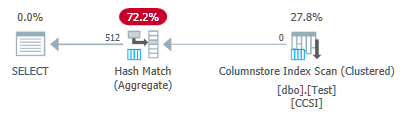The plan xml contains `ActualLocallyAggregatedRows="32256"` in the runtime information for the index scan.

## Test 2: No pushdown, 12-bit Impure Keys

This test changes the `@values` parameter to 1025, keeping `@dupes` at 63. This gives a table of 64,575 rows, with 1,025 distinct values in column `c2` running from 0 to 1024 inclusive. Each value in `c2` is duplicated 63 times.

SQL Server stores the `c2` column data using:

• Dictionary encoding since there are a significant number of duplicated values.
• No RLE. The number of duplicates (63) per value does not reach the threshold of 64 required for RLE.
• Bit-packed with size 12. The 1,025 distinct dictionary entries will not quite fit in 10 bits (2^10 = 1,024). They would fit in 11 bits but SQL Server does not support that bit-packing size as previously mentioned. The next smallest size is 12 bits. Using 64-bit units with hard borders for bit-packing, no more 11-bit subunits could fit in 64 bits than 12-bit subunits would. Either way, 5 subunits will fit in a 64-bit unit.

The `DBCC CSINDEX` output confirms the above analysis:

Segment Attributes:

Version = 1 encodingType = 2 hasNulls = 0
BaseId = -1 Magnitude = -1.000000e+000 PrimaryDictId = 0
SecondaryDictId = -1 MinDataId = 0 MaxDataId = 1024
NullValue = -1 OnDiskSize = 104400 RowCount = 64575

Lob type = 3 RLE Array Count (In terms of Native Units) = 2
RLE Array Entry Size = 8

RLE Data:

Index = 0 Bitpack Array Index = 0 Count = 64575
Index = 1 Value = 0 Count = 0

Bitpack Entry Size = 12 Bitpack Unit Count = 12915 Bitpack MinId = 3
Bitpack DataSize = 103320
Bitpack Data:

Unit 0 SubUnit 0 = 767
Unit 0 SubUnit 1 = 510
Unit 0 SubUnit 2 = 254
Unit 0 SubUnit 3 = 1021
Unit 0 SubUnit 4 = 765

Unit 1 SubUnit 0 = 507
Unit 1 SubUnit 1 = 250
Unit 1 SubUnit 2 = 1019
Unit 1 SubUnit 3 = 761
Unit 1 SubUnit 4 = 505

Since the impure grouping keys have a size over 10, we expect grouped aggregate pushdown not to work here. This is confirmed by the execution plan showing zero rows locally aggregated at the Columnstore Index Scan operator: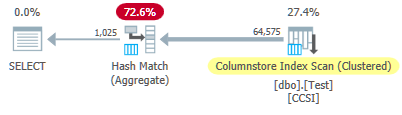All 64,575 rows are emitted (in batches) by the Columnstore Index Scan and aggregated in batch mode by the Hash Match Aggregate operator. The `ActualLocallyAggregatedRows` attribute is missing from the xml plan runtime information for the index scan.

## Test 3: Pushdown, Pure Keys

This test changes the `@dupes` parameter from 63 to 64 to allow RLE. The `@values` parameter is changed to 16,384 (the maximum for the total number of rows to still fit in a single row group). The exact number chosen for `@values` is not important — the point is to generate 64 duplicates of each unique value so RLE can be used.

SQL Server stores the `c2` column data using:

• Dictionary encoding due to the duplicated values.
• RLE. Used for every distinct value since each meets the threshold of 64.
• No bit-packed data. If there were any, it would use size 16. Size 12 is not large enough (2^12 = 4,096 distinct values) and size 21 would be wasteful. The 16,384 distinct values would fit in 14 bits but, as before, no more of these can fit in a 64-bit unit than 16-bit subunits.

The `DBCC CSINDEX` output confirms the above (only a few RLE entries and bookmarks shown for space reasons):

Segment Attributes:

Version = 1 encodingType = 2 hasNulls = 0
BaseId = -1 Magnitude = -1.000000e+000 PrimaryDictId = 0
SecondaryDictId = -1 MinDataId = 0 MaxDataId = 16383
NullValue = -1 OnDiskSize = 131648 RowCount = 1048576

Lob type = 3 RLE Array Count (In terms of Native Units) = 16385
RLE Array Entry Size = 8

RLE Data:

Index = 0 Value = 3 Count = 64
Index = 1 Value = 1538 Count = 64
Index = 2 Value = 3072 Count = 64
Index = 3 Value = 4608 Count = 64
Index = 4 Value = 6142 Count = 64

Index = 16381 Value = 8954 Count = 64
Index = 16382 Value = 10489 Count = 64
Index = 16383 Value = 12025 Count = 64
Index = 16384 Value = 0 Count = 0

Bookmark Count = 65 Bookmark Distance = 16384 Bookmark Size = 520

Bookmark Data:

Position = 0 Index = 64
Position = 512 Index = 16448
Position = 1024 Index = 32832

Position = 31744 Index = 1015872
Position = 32256 Index = 1032256
Position = 32768 Index = 1048577

Bitpack Entry Size = 16 Bitpack Unit Count = 0 Bitpack MinId = 3
Bitpack DataSize = 0

Since the grouping keys are pure (RLE is used), grouped aggregate pushdown is expected here. The execution plan confirms this by showing all rows locally aggregated at the Columnstore Index Scan operator: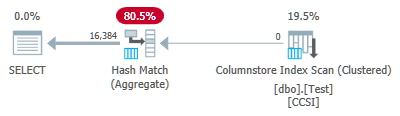The plan xml contains `ActualLocallyAggregatedRows="1048576"` in the runtime information for the index scan.

## Test 4: 10-bit Impure Keys

This test sets `@values` to 1024 and `@dupes` to 63, giving a table of 64,512 rows, with 1,024 distinct values in column `c2` with values from 0 to 1,023 inclusive. Each value in `c2` is duplicated 63 times.

Most importantly, the b-tree clustered index is now created on column `c1` instead of column `c2`. The clustered columnstore still replaces the b-tree clustered index. This is the changed part of the script:

```-- Note column c1 now!
CREATE CLUSTERED INDEX CCSI ON dbo.Test (c1);
GO
CREATE CLUSTERED COLUMNSTORE INDEX CCSI
ON dbo.Test
WITH (MAXDOP = 1, DROP_EXISTING = ON);```

SQL Server stores the `c2` column data using:

• Dictionary encoding due to the duplicates.
• No RLE. The number of duplicates (63) per value does not reach the threshold of 64 required for RLE.
• Bit-packing with size 10. The 1,024 distinct dictionary entries exactly fit in 10 bits (2^10 = 1,024). Six subunits of 10 bits each can be stored in each 64-bit unit.

The `DBCC CSINDEX` output is:

Segment Attributes:

Version = 1 encodingType = 2 hasNulls = 0
BaseId = -1 Magnitude = -1.000000e+000 PrimaryDictId = 0
SecondaryDictId = -1 MinDataId = 0 MaxDataId = 1023
NullValue = -1 OnDiskSize = 87096 RowCount = 64512

Lob type = 3 RLE Array Count (In terms of Native Units) = 2
RLE Array Entry Size = 8

RLE Data:

Index = 0 Bitpack Array Index = 0 Count = 64512
Index = 1 Value = 0 Count = 0

Bitpack Entry Size = 10 Bitpack Unit Count = 10752 Bitpack MinId = 3
Bitpack DataSize = 86016
Bitpack Data:

Unit 0 SubUnit 0 = 766
Unit 0 SubUnit 1 = 509
Unit 0 SubUnit 2 = 254
Unit 0 SubUnit 3 = 1020
Unit 0 SubUnit 4 = 764
Unit 0 SubUnit 5 = 506

Unit 1 SubUnit 0 = 250
Unit 1 SubUnit 1 = 1018
Unit 1 SubUnit 2 = 760
Unit 1 SubUnit 3 = 504
Unit 1 SubUnit 4 = 247
Unit 1 SubUnit 5 = 1014

Since the impure grouping keys use a size less than or equal to 10, we would expect grouped aggregate pushdown to work here. But that is not what happens. The execution plan shows 54,612 of the 64,512 rows were aggregated at the Hash Match Aggregate operator: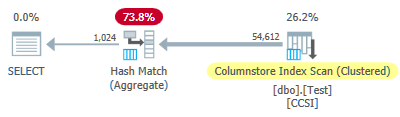The plan xml contains `ActualLocallyAggregatedRows="9900"` in the runtime information for the index scan. This means grouped aggregate pushdown was used for 9,900 rows, but not used for the other 54,612!

### The feedback mechanism

SQL Server started off using grouped aggregate pushdown for this execution because the impure grouping keys met the 10-bits-or-less criteria. This lasted for a total of 11 batches (of 900 rows each = 9,900 rows total). At that point, a feedback mechanism measuring the effectiveness of grouped aggregate pushdown decided it was not working out, and turned it off. The remaining batches were all processed with pushdown disabled.

The feedback essentially compares the number of rows aggregated against the number of groups produced. It starts off with a value of 100 and is adjusted at the end of each pushdown output batch. If the value drops to 10 or below, pushdown is disabled for the current grouping operation.

The ‘pushdown benefit measure’ is reduced more or less depending on how badly the pushed-down aggregation effort is going. If there are fewer than 8 rows per grouping key on average in the output batch, the current benefit value is reduced by 22%. If there are more than 8 but fewer than 16, the metric is reduced by 11%.

On the other hand, if things improve, and 16 or more rows per grouping key are subsequently encountered for an output batch, the metric is reset to 100, and continues to be adjusted as partial aggregate batches are produced by the scan.

The data in this test was presented in a particularly unhelpful order for pushdown due to the original b-tree clustered index on column `c1`. When presented this way, the values in column `c2` begin at 0 and increment by 1 until they reach 1,023, then they start the cycle again. The 1,023 distinct values are more than enough to ensure each 900-row output batch contains only one partially aggregated row for each key. This is not a happy state.

If there had been 64 duplicates per value instead of 63, SQL Server would have considered sorting by `c2` while building the columnstore index, and so produced RLE compression. As it is, the the 22% penalty kicks in after every batch. Starting at 100 and using the same round-up integer arithmetic, the sequence of metric values goes:

```-- @metric := FLOOR(@metric * 0.78 + 0.5);
-- 100, 78, 61, 48, 37, 29, 23, 18, 14, 11, *9*```

The eleventh batch reduces the metric to 10 or below, and pushdown is disabled. The 11 batches of 900 rows account for the 9,900 locally aggregated rows shown in the execution plan.

### Variation with 900 distinct values

The same behaviour can be seen in test 4 with as few as 901 distinct values, assuming the rows happen to be presented in the same unhelpful order.

Changing the `@values` parameter to 900 while keeping everything else the same has a dramatic effect on the execution plan: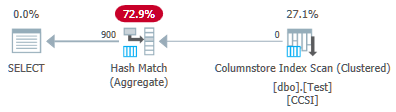Now all 900 groups are aggregated at the scan! The xml plan properties show `ActualLocallyAggregatedRows="56700"`. This because grouped aggregate pushdown maintains 900 grouping keys and partial aggregates in a single batch. It never encounters a new key value not in the batch, so there is no reason to start a fresh output batch.

Only ever producing one batch means the feedback mechanism never gets chance to reduce the “pushdown benefit measure” to the point where grouped aggregate pushdown is disabled. It never would anyway, since the pushdown is very successful — 56,700 rows for 900 grouping keys is 63 per key, well above the threshold for benefit measure reduction.

## Extended Event

There is very little information available in execution plans to help determine why grouped aggregation pushdown was either not tried, or was not successful. There is, however, an Extended Event named `query_execution_dynamic_push_down_statistics` in the execution category of the Analytic channel.

It provides the following Event Fields:

`rows_not_pushed_down_due_to_encoding`

Description: Number of rows not pushed to scan because of the the total encoded key length.

This identifies impure data over the 10-bit limit as shown in test 2.

`rows_not_pushed_down_due_to_possible_overflow`

Description: Number of rows not pushed to scan because of a possible overflow

`rows_not_pushed_down_due_to_pushdown_disabled`

Description: Number of rows not pushed to scan (only) because dynamic pushdown was disabled

This occurs when the pushdown benefit measure drops below 10 as described in test 4.

`rows_pushed_down_in_thread`

Description: Number of locally aggregated rows in thread

This corresponds with the value for ‘locally aggregated rows’ shown in execution plans.

Note: No event is recorded if grouped aggregation pushdown is specifically disabled using trace flag 9373. All types of pushdown to a nonclustered columnstore index can be specifically disabled with trace flag 9386. All types of pushdown activity can be disabled with trace flag 9354.